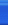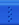# penven博客园首页新随笔联系订阅管理/*
* php 四种排序算法的时间与内置的sort排序比较
* 3000个元素，四种算法的排序所用的时间比较
* 冒泡排序 857.98192024231ms
* 选择排序 903.74493598938ms
* 插入排序 296.8270778656ms
* 快速排序 15.607833862305ms
* sort排序 0.95200538635254ms
* 归并排序 14.61386680603ms
* */

/*
* @param 冒泡排序
* 它重复地走访过要排序的数列，一次比较两个元素，如果他们的顺序错误就把他们交换过来。
* 走访数列的工作是重复地进行直到没有再需要交换，也就是说该数列已经排序完成。
* */
function BubbleSort($arr) {$len = count($arr); //设置一个空数组 用来接收冒出来的泡 //该层循环控制 需要冒泡的轮数 for ($i = 1; $i <$len; $i++) {$flag = false;    //本趟排序开始前，交换标志应为假
//该层循环用来控制每轮 冒出一个数 需要比较的次数
for ($k = 0;$k < $len -$i; $k++) { //从小到大排序 if ($arr[$k] >$arr[$k + 1]) {$tmp = $arr[$k + 1];
$arr[$k + 1] = $arr[$k];
$arr[$k] = $tmp;$flag = true;
}
}
if(!$flag) return$arr;
}
}

/*
* @param 选择排序法
* 每一次从待排序的数据元素中选出最小（或最大）的一个元素，存放在序列的起始位置，直到全部待排序的数据元素排完。
* 选择排序是不稳定的排序方法（比如序列[5， 5， 3]第一次就将第一个与交换，导致第一个5挪动到第二个5后面）
* */
function selectSort($array){$temp = 0;
for($i = 0;$i < count($array) - 1;$i++){
$minVal =$array[$i]; //假设$i就是最小值
$minValIndex =$i;
for($j =$i+1;$j < count($array);$j++){ if($minVal > $array[$j]){ //从小到大排列
$minVal =$array[$j]; //找最小值$minValIndex = $j; } }$temp = $array[$i];
$array[$i] = $array[$minValIndex];
$array[$minValIndex] = $temp; } } /* * 插入排序法 * 每步将一个待排序的纪录，按其关键码值的大小插入前面已经排序的文件中适当位置上，直到全部插入完为止。 * 算法适用于少量数据的排序，时间复杂度为O(n^2)。是稳定的排序方法。 * */ function insertSort($array){ //从小到大排列
//先默认$array，已经有序，是有序表 for($i = 1;$i < count($array);$i++){$insertVal = $array[$i]; //$insertVal是准备插入的数$insertIndex = $i - 1; //有序表中准备比较的数的下标 while($insertIndex >= 0 && $insertVal <$array[$insertIndex]){$array[$insertIndex + 1] =$array[$insertIndex]; //将数组往后挪$insertIndex--; //将下标往前挪，准备与前一个进行比较
}
if($insertIndex + 1 !==$i){
$array[$insertIndex + 1] = $insertVal; } } } /* * 快速排序法 * 通过一趟排序将要排序的数据分割成独立的两部分，其中一部分的所有数据都比另外一部分的所有数据都要小， * 然后再按此方法对这两部分数据分别进行快速排序，整个排序过程可以递归进行，以此达到整个数据变成有序序列。 * */ function quickSort($array){
if(!isset($array)) return$array;
$mid =$array; //获取一个用于分割的关键字，一般是首个元素
$leftArray = array();$rightArray = array();
foreach($array as$v){
if($v >$mid)
$rightArray[] =$v; //把比$mid大的数放到一个数组里 if($v < $mid)$leftArray[] = $v; //把比$mid小的数放到另一个数组里
}
$leftArray = quickSort($leftArray); //把比较小的数组再一次进行分割
$leftArray[] =$mid; //把分割的元素加到小的数组后面，不能忘了它哦
$rightArray = quickSort($rightArray); //把比较大的数组再一次进行分割
return array_merge($leftArray,$rightArray); //组合两个结果
}

/*
* 归并排序
* 归并排序是指将两个或两个以上有序的数列（或有序表），合并成一个仍然有序的数列（或有序表）。
* 这样的排序方法经常用于多个有序的数据文件归并成一个有序的数据文件。
* */
function mergeSort(&$arr) {$len = count($arr);//求得数组长度 mSort($arr, 0, $len-1); return$arr;
}
//实际实现归并排序的程序
function mSort(&$arr,$left, $right) { if($left < $right) { //说明子序列内存在多余1个的元素，那么需要拆分，分别排序，合并 //计算拆分的位置，长度/2 去整$center = floor(($left+$right) / 2);
//递归调用对左边进行再次排序：
mSort($arr,$left, $center); //递归调用对右边进行再次排序 mSort($arr, $center+1,$right);
//合并排序结果
mergeArray($arr,$left, $center,$right);
}
}
//将两个有序数组合并成一个有序数组
function mergeArray(&$arr,$left, $center,$right) {
//设置两个起始位置标记
$a_i =$left;
$b_i =$center+1;
while($a_i<=$center && $b_i<=$right) {
//当数组A和数组B都没有越界时
if($arr[$a_i] < $arr[$b_i]) {
$temp[] =$arr[$a_i++]; } else {$temp[] = $arr[$b_i++];
}
}
//判断 数组A内的元素是否都用完了，没有的话将其全部插入到C数组内：
while($a_i <=$center) {
$temp[] =$arr[$a_i++]; } //判断 数组B内的元素是否都用完了，没有的话将其全部插入到C数组内： while($b_i <= $right) {$temp[] = $arr[$b_i++];
}

//将$arrC内排序好的部分，写入到$arr内：
for($i=0,$len=count($temp);$i<$len;$i++) {
$arr[$left+$i] =$temp[$i]; } }$a = array_rand(range(1,10000), 3000); //生成3000个元素的随机数组
shuffle($a); //打乱数组的顺序$t1 = microtime(true);
BubbleSort($a); //冒泡排序$t2 = microtime(true);
echo "冒泡排序用时：".(($t2-$t1)*1000).'ms'."\n";

$t3 = microtime(true); selectSort($a); //选择排序
$t4 = microtime(true); echo "选择排序用时：".(($t4-$t3)*1000).'ms'."\n";$t5 = microtime(true);
insertSort($a); //插入排序$t6 = microtime(true);
echo "插入排序用时：".(($t6-$t5)*1000).'ms'."\n";

$t7 = microtime(true); quickSort($a); //快速排序
$t8 = microtime(true); echo "快速排序用时：".(($t8-$t7)*1000).'ms'."\n";$t9 = microtime(true);
sort($a);$t10 = microtime(true);
echo "sort排序用时：".(($t10-$t9)*1000)."ms"."\n";

$t11 = microtime(true); mergeSort($a);
$t12 = microtime(true); echo "归并排序用时：".(($t12-\$t11)*1000)."ms";

从时间上来看，快速排序和归并排序在时间上比较有优势，但是也比不上sort排序，归并排序比较占用内存！

posted on 2017-01-16 15:30  penven  阅读(14889)  评论(0编辑  收藏  举报